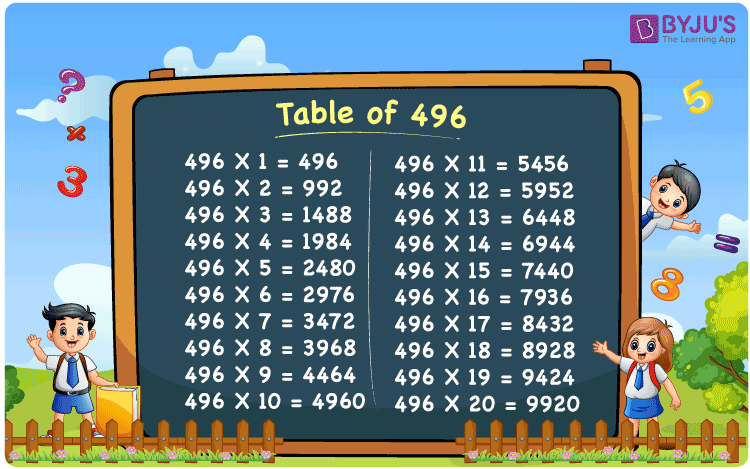Checkout JEE MAINS 2022 Question Paper Analysis : Checkout JEE MAINS 2022 Question Paper Analysis :

# Table of 496

The Multiplication Table for 496 is nothing more than the repetitive addition of the number 496. 496 is an even number that is divisible by 2. Note that 496 is also divisible by 4. Observe that all the multiples of 496 are also divisible by 2 and 4. You can read more on Divisibility rules.

## Table of 496 Chart## What is the 496 Times Table?

In the table of 496, we are repeatedly adding 496 multiple times. And the table below gives a repetitive addition 10 times for the number 496.

Given below is the multiplication table of 496 done 20 times. The below table helps you with multiplication problems that require the tables of 496.

Solved Example on Table of 496

Find the value of y: 496 x y = 7440.

Solution: y = 15. Given 496 x y = 7440y = 7440 / 496 = 15.

 496 × 1 = 496 496 496 × 2 = 992 496 + 496 = 992 496 × 3 = 1488 496 + 496 + 496 = 1488 496 × 4 = 1984 496 + 496 + 496 + 496 = 1984 496 × 5 = 2480 496 + 496 + 496 + 496 + 496 = 2480 496 × 6 = 2976 496 + 496 + 496 + 496 + 496 + 496 = 2976 496 × 7 = 3472 496 + 496 + 496 + 496 + 496 + 496 + 496 = 3472 496 × 8 = 3968 496 + 496 + 496 + 496 + 496 + 496 + 496 + 496 = 3968 496 × 9 = 4464 496 + 496 + 496 + 496 + 496 + 496 + 496 + 496 + 496 = 4464 496 × 10 = 4960 496 + 496 + 496 + 496 + 496 + 496 + 496 + 496 + 496 + 496 = 4960 496 × 1 = 496 496 × 2 = 992 496 × 3 = 1488 496 × 4 = 1984 496 × 5 = 2480 496 × 6 = 2976 496 × 7 = 3472 496 × 8 = 3968 496 × 9 = 4464 496 × 10 = 4960 496 × 11 = 5456 496 × 12 = 5952 496 × 13 = 6448 496 × 14 = 6944 496 × 15 = 7440 496 × 16 = 7936 496 × 17 = 8432 496 × 18 = 8928 496 × 19 = 9424 496 × 20 = 9920